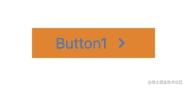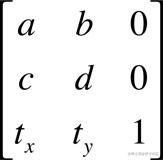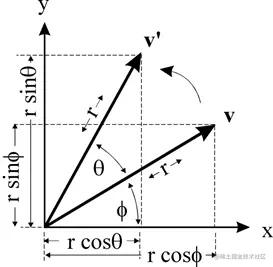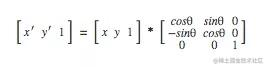Transform的那点事####实现原理

struct CGAffineTransform {
CGFloat a, b, c, d;
CGFloat tx, ty;
};x' = ax+cy+tx

y' = bx+dy+ty

1.平移

/* Return a transform which translates by (tx, ty)':
t' = [ 1 0 0 1 tx ty ] */

CG_EXTERN CGAffineTransform CGAffineTransformMakeTranslation(CGFloat tx,
CGFloat ty) CG_AVAILABLE_STARTING(10.0, 2.0);

2.缩放

/* Return a transform which scales by (sx, sy)':
t' = [ sx 0 0 sy 0 0 ] */

CG_EXTERN CGAffineTransform CGAffineTransformMakeScale(CGFloat sx, CGFloat sy)
CG_AVAILABLE_STARTING(10.0, 2.0);

3. 旋转

/* Return a transform which rotates by `angle' radians:
t' = [ cos(angle) sin(angle) -sin(angle) cos(angle) 0 0 ] */

CG_EXTERN CGAffineTransform CGAffineTransformMakeRotation(CGFloat angle)
CG_AVAILABLE_STARTING(10.0, 2.0);x=rcosϕ, y=rsinϕ

x′=rcos(θ+ϕ), y′=rsin(θ+ϕ)

x′=rcosθcosϕ−rsinθsinϕ

y′=rsinθcosϕ+rcosθsinϕ

x′=xcosθ−ysinθ

y′=xsinθ+ycosθQuartz 2D绘图模型有两种空间，用户空间(user space)和设备空间(device space)。用户空间表示当前需绘制的文档页(document page),设备空间表示原始分辨率的设备。Quartz 2D使用一个变换矩阵CTM(current transformation matrix)将用户空间映射到设备空间。CTM存储在图形上下文( graphics context)中，初始值为identity matrix。在绘制过程中可进行修改。 修改当前CTM的API有CGContextRotateCTM CGContextScaleCTM CGContextTranslateCTM分别用于旋转，缩放，平移。Rotate是以原点为圆心旋转,Quartz创建的图形上下文旋转圆心为左下角，角度值正数为逆时针旋转，负数为顺时针旋转;而UIKit创建的图像上下文旋转圆心为左上角,角度值正数为顺时针旋转，负数为逆时针旋转。

问题解答

1. 使用UIView(UILabel + UIImage)
2. 使用UIControl(UILabel + UIImage)
3. 使用UIButton, 手动更改edgeinset
4. 使用UIButton, 手动更改frame
5. ...

self.transform = CGAffineTransformMakeScale(-1.0, 1.0);
self.titleLabel.transform = CGAffineTransformMakeScale(-1.0, 1.0);
self.imageView.transform = CGAffineTransformMakeScale(-1.0, 1.0);